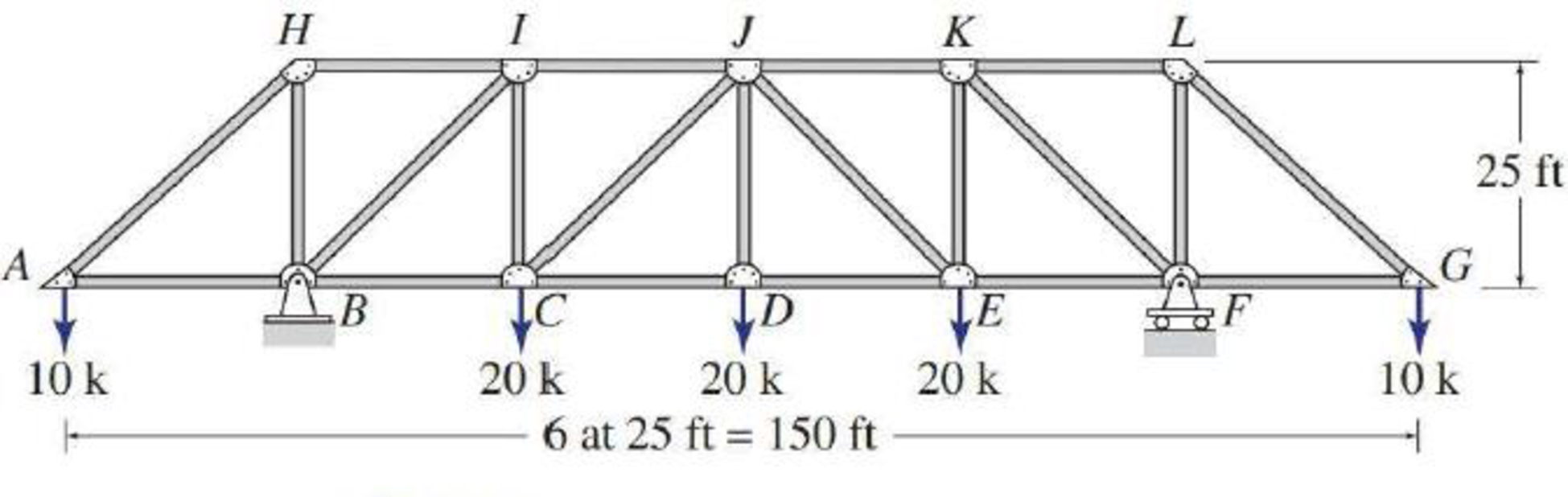# 4.6 through 4.28 Determine the force in each member of the truss shown by the method of joints. FIG. P4.15

#### Solutions

Chapter
Section
Chapter 4, Problem 15P
Textbook Problem
60 views

## 4.6 through 4.28 Determine the force in each member of the truss shown by the method of joints.FIG. P4.15To determine

Find the forces in the members of the truss by the method of joints.

### Explanation of Solution

Given information:

Apply the sign conventions for calculating reactions, forces and moments using the three equations of equilibrium as shown below.

• For summation of forces along x-direction is equal to zero (Fx=0), consider the forces acting towards right side as positive (+) and the forces acting towards left side as negative ().
• For summation of forces along y-direction is equal to zero (Fy=0), consider the upward force as positive (+) and the downward force as negative ().
• For summation of moment about a point is equal to zero (Matapoint=0), consider the clockwise moment as negative and the counter clockwise moment as positive.

Method of joints:

The negative value of force in any member indicates compression (C) and the positive value of force in any member indicates Tension (T).

Calculation:

Consider the forces in the member AB, BC, CD, DE, EF, FG, AH, HI, IJ, JK, KL, LG, BH, BI, CI, CJ, FL, FK, EK, EJ, DJ are FAB,FBC,FCD,FDE,FEF,FFG,FAH,FHI,FIJ,FJK,FKL,FLG,FBH, FBI, FCI, FCJ, FFL, FFK, FEK, FEJ, FDJ.

Show the free body diagram of the truss as shown in Figure 1.

Refer Figure 1.

Consider the horizontal and vertical reactions at B are Bx and By.

Consider the vertical reaction at F is Fy.

By=Fy=10+20+20+20+102By=Fy=40k

Take the sum of the forces in the horizontal direction as zero.

Fx=0Bx=0

Consider the angle made by each of the inclined member with the horizontal is denoted by θ.

Calculate the value of the angle θ as follows:

tanθ=2525θ=tan1(1)θ=45°

Show the joint A as shown in Figure 2.

Refer Figure 2.

Find the forces in the member AB and AH as follows:

For Equilibrium of forces,

Fy=0FAHsin(45°)=10FAH=14.14k(T)

Fx=0FAHcos(45°)+FAB=0FAB=FAHcos(45°)FAB=14.14cos(45°)FAB=10k(C)

Show the joint H as shown in Figure 3.

Refer Figure 3.

Find the forces in the member HI and HB as follows:

For Equilibrium of forces,

Fx=0FHI=FHAsin(45°)FHI=14.14sin(45°)FHI10k(T)

Fy=0FHB=FAHcos(45°)FHB=14.14cos(45°)FHB10k(C)

Show the joint B as shown in Figure 4.

Refer Figure 4.

Find the forces in the member BI and BC as follows:

For Equilibrium of forces,

Fy=040+FBH+FBIsin(45°)=0FBI=(40FBHsin(45°))FBI=(40(10)sin(45°))FBI=42.43k(C)

Fx=0FBA+FBC+FBIcos45°=0FBC=FBAFBIcos45°FBC=(10)(42.43)cos45°FBC=20k(T)

Show the joint I as shown in Figure 5.

Refer Figure 5.

Find the forces in the member IC and IJ as follows:

For Equilibrium of forces,

Fy=0FICFIBcos45°=0FIC=FIBcos45°FIC=(42

### Still sussing out bartleby?

Check out a sample textbook solution.

See a sample solution

#### The Solution to Your Study Problems

Bartleby provides explanations to thousands of textbook problems written by our experts, many with advanced degrees!

Get Started

Find more solutions based on key concepts
Identify the size and the type of the given matrices. Denote whether the matrix is a square, a column, a diagon...

Engineering Fundamentals: An Introduction to Engineering (MindTap Course List)

Briefly define the term cutting speed.

Precision Machining Technology (MindTap Course List)

How many records does the file contain? How many fields are there per record?

Database Systems: Design, Implementation, & Management

Bad Directions You are driving to your friends house and are using your smartphone for directions. While approa...

Enhanced Discovering Computers 2017 (Shelly Cashman Series) (MindTap Course List)

If your motherboard supports ECC DDR3 memory, can you substitute non-ECC DDR3 memory?

A+ Guide to Hardware (Standalone Book) (MindTap Course List)